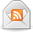# How to Calculate Compound Interest

Posted by Don on March 18, 2015

Compound interest is the most powerful force in the universe, which was supposedly said by Albert Einstein, though there is no proof that he actually said this. Nonetheless, compound interest is an amazing thing.

Previously, I talked about how to calculate simple interest. Now, I am going to show you how to calculate compound interest. But before we get into the calculation, we have to understand what compound interest is.

### What Is Compound Interest?

As I pointed out in how to calculate interest, when you earn simple interest, you only earn interest on your principal amount of money. With compound interest, all of this changes.

Compound interest allows you to earn interest on your interest. You read that right – you earn interest on both your principal and the interest you earn. Let’s look at an example. Let’s say you have \$100 and you are earning 10% interest. If you are earning simple interest on your \$100, at the end of the year, you have \$110. But, if the interest you earn is compound interest and it compounds monthly, you have \$110.47 at year end. You earned an extra \$0.47 because of the effects of compounding.

### How to Calculate Compound Interest

To see how this works, we need to look at the formula for compound interest. Here is the formula for compound interest:

Let’s plug in some numbers so you can see how this works. Let’s assume this time we have \$1,000 and we are earning 5% interest on our money. Interest is compounded monthly and we leave the money alone for 3 years. How much money do we end up with? Here is the formula with our numbers:

\$1,000 (1 + 0.05/12)^12(3)

The answer is \$1,161.47. The great thing about compound interest is, the more frequently you compound, the more interest you earn, up to a point. If we take the same example we just used and instead of compounding monthly, we compounded interest daily, we would have \$1,161.82. Because of the increased frequency of compounding, we earned an additional \$0.35. I know that doesn’t seem like much, but if we had more money saved, we would have earned more money from compound interest. Likewise, if we had more time, meaning more compounding periods, we would end up with more money as well.

### The Limit of Compound Interest

Remember above how I said that the more frequently you compound the more interest you earn, to a point? Let’s look at an example for proof. In the above example, we had \$1,000 earning 5% interest for 3 years and compounding daily. We ended up with \$1,161.82. If we were to compound hourly instead of daily, over the course of three years, we would earn an additional \$0.01. That’s it. But don’t confuse the frequency of compounding with years. When I talk about frequency of compounding, I am only talking about how often interest is compounded – quarterly, monthly, daily, etc. Ideally you want daily compounding and you want to let compound interest work for years.

### Final Thoughts

The power of compound interest is great. The more money you save and the longer you leave it alone to grow, the more compound interest will work its magic. But just like with anything in life, the power of compound interest won’t make you rich overnight. It takes time to work its magic and it needs you to be smart with your money and continue to save more and more. If you can do this, you will experience the power of compound interest.

### More Financial Calculations